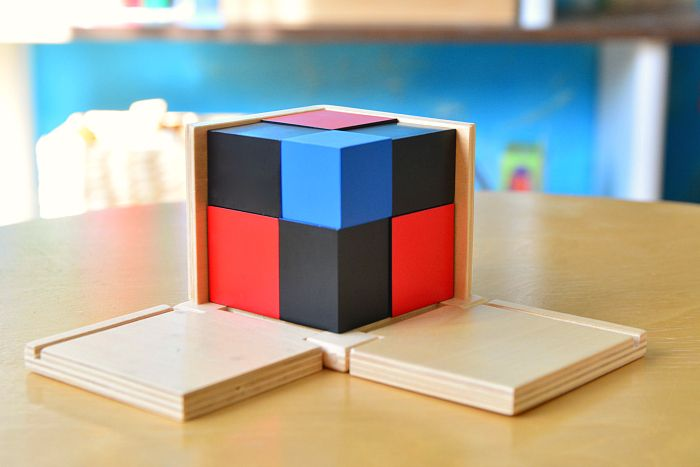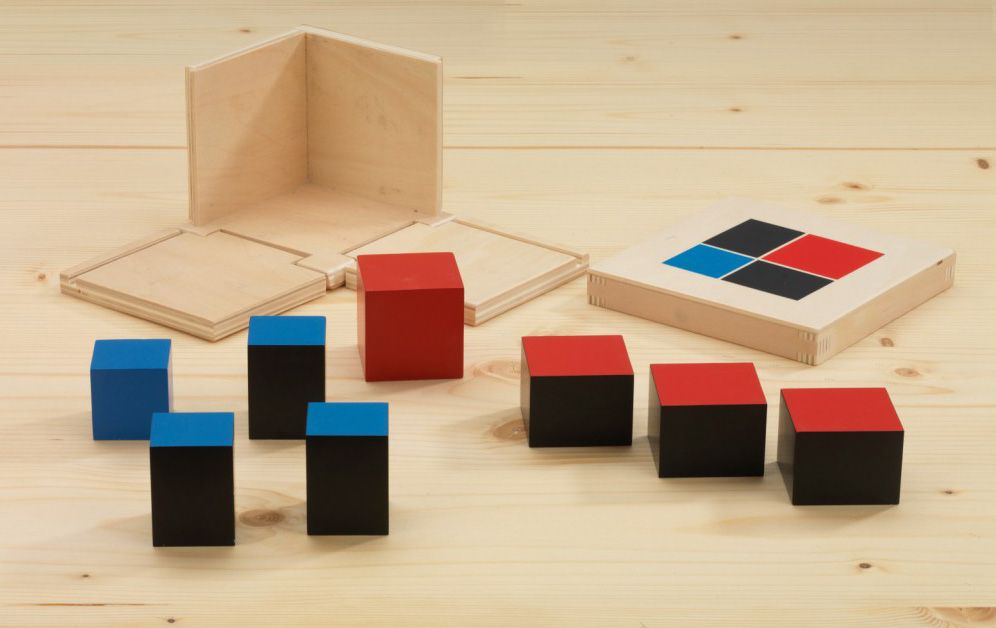Nirmala V Mani

## Binomial cube

The Montessori binomial cube is a valuable mathematical tool used to teach children about binomial expansions and the concept of cubes. The cube is constructed of 27 small cubes, each face of the large cube represents a different term in the expansion of (a + b)^3. The purpose of this material is to aid children in visualizing the concept of binomial expansions and to grasp the relationship between the different terms.

### Objectives

• To prepare the child for later work in Mathematics, especially algebra
• To aid the child’s fine motor
• Eye- hand co-ordination
• Concentration### Presentation

The blocks are colour coded and are different sizes. They fit together to create a binomial pattern. The Binomial Cube is a concrete representation of the algebraic formula (a+b)3. The Binomial Cube is a sensorial material and the purpose is not to teach maths, but to provide a challenge for a child’s ability to find patterns and relationships. It is presented like a three-dimensional puzzle.It is amazing to see an abstract concept taught to young children in a concrete, hands-on way. It introduces abstract math concepts to the young child without them even knowing it. The early sensorial experience with the cube provides learning that will assist the child when revisiting algebra at a much later age. It lays the foundation for a much deeper understanding of maths.

Through demonstration, the child is shown to systematically deconstruct then reconstruct the cube. The child uses their senses of touch and sight, using size and colour to build the cube. The child also uses their developing visual perception, concentration and problem-solving skills.Excercises

Exercises using the binomial cube can include finding the number of small cubes needed to make a specific term in the expansion, or matching a specific term in the expansion to the corresponding face of the large cube. The child can also be asked to construct different shapes using the small cubes and identify the corresponding term in the expansion. As the child becomes more familiar with the material and the concept of binomial expansions, more advanced exercises can be introduced, such as expanding (a + b)^4 or (a + b)^5.

In conclusion, the Montessori Binomial Cube is a valuable tool for teaching children about binomial expansions and the concept of cubes in a hands-on, interactive way. It encourages exploration, discovery, and a deeper understanding of mathematical concepts.